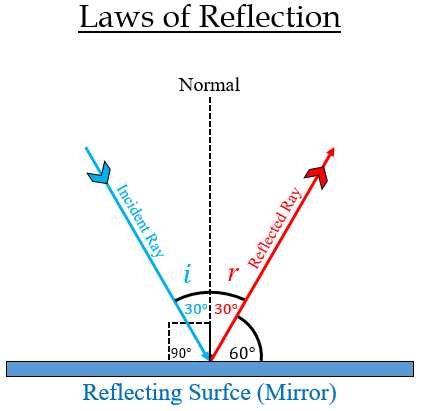# A ray of light strikes a plane mirror such that its angle of incidence is 30°. What angle does the reflected ray make with the mirror surface?

Here, the angle of incidence (the angle between the incident ray and the normal) = 30°.

Therefore, the angle of reflection (the angle between the reflected ray and the normal) is also = 30°.

According to the law of reflection, which states that the angle of incidence is equal to the angle of reflection.

Now, the angle from the mirror surface (the angle between the reflected ray and the mirror) = 90° $-$ angle of reflection = 90° $-$ 30°= 60°

Thus, the angle of the reflected ray with the mirror surface 60°.

Image is posted for reference only.Updated on: 10-Oct-2022

602 Views